Accueil > Un parcours historique... de la boussole à la Fée électricité > Des lois pour le courant : Ampère, Ohm et quelques autres... > Ampère's Force Law: An Obsolete Formula?

# Ampère's Force Law: An Obsolete Formula?Français

By Christine Blondel and Bertrand Wolff
Translated by Andrew Butrica

It is not uncommon to find in the science of the past some works that never were deemed as being invalid yet have disappeared from modern science. They might have been overturned by subsequent work judged to be more effective, or were abandoned because they were considered to be of little interest, or were discredited for nonscientific reasons, or simply were forgotten. Their status remained undecided. At any moment, though, scientists can take up such an unclaimed work for different reasons. Such is the case of Ampère's force.

Ampère's electrodynamic theory is based on the existence of a force between two current elements, and the mathematical expression of this force implies a repulsion between two aligned elements. However, this interaction between collinear elements disappeared with the elemental force of Grassmann (1845), a force that forms now an integral part of the modern theory of electromagnetism.

In order to decide between Ampère and Grassmann expressions of the electrodynamic force, one can look for experiments relying on the existence, or the non-existence, of a repulsion between collinear elements of an electric circuit. Nonetheless, a direct verification of Ampère's formula and its application to the particular case of collinear elements is not possible. Indeed, one cannot isolate current elements within an electric circuit. Ampère thought, though, to have an experimental proof for the repulsion between collinear elements. We will discuss various experiments proposed at the end of the twentieth century in the continuation of Ampère's experiment and likely to demonstrate the existence of this force. The interpretation of these experiments is still controversial.

From a theoretical point of view, the compatibility between the Maxwell-Lorentz theory and Ampère's force also is a subject of debate.

Finally, for some, defending Ampère's force is part of a larger project that consists of developing - following Weber and Neumann - a “Newtonian” alternative to Maxwell's theory, using only interactions at a distance between material elements without recourse to an electromagnetic field. This project may seem foolhardy and based on tenuous foundations, while Maxwell's theory, associated with Einstein's relativity, constitutes a major pillar of modern physics.

We conclude, nonetheless, following Maxwell himself, that it is desirable for the very life of science to leave open a number of paths: “"“It is a good thing to have two ways of looking at a subject, and to admit that there are two ways of looking at it."” (The Scientific Papers of James Clerk Maxwell, t. 1, p. 208)

### 1873: “A formula from which all phenomena may be deduced...”

According to Maxwell himself, “Ampère's theory "is summed up in a formula from which all the phenomena may be deduced, and which must always remain the cardinal formula of electro-dynamics."” (A Treatise on Electricity and Magnetism, 1873, t. 2, p. 175) .

This fundamental formula of Ampère expresses the force exerted by one infinitesimal current element ids on another infinitesimal current element i'ds', placed at a distance r from each other and whose relative positions are defined by the three angles α, β and γ [See the page In Search of a Newtonian Law of Electrodynamics].Ampère's elementary force between ids and i'ds':i i' ds ds' (sinα sinβ cosγ - ½ cosα cosβ)/ r2

Fig. 1. The current element ds, located at point A, is situated in the plane P; element ds' located at point B is in the plane Q.

From this formula Ampère could calculate, by a double integration, all the interactions between real circuits. Applying this method to the small circular currents, which he supposed to exist in the interior of magnets, he obtained the laws of interaction between two magnets or between a magnet and a current. Relying on this formula one can, as Maxwell wrote, “deduce all phenomena.”

### 1958: A formula that “no longer serves any purpose”

In 1958, Edmond Bauer, who had collaborated with Paul Langevin on his theory of magnetism, reworking Ampère's fundamental ideas, reissued Ampère's great synthesis, Mathematical Theory of Electrodynamic Phenomena Uniquely Deduced from Experiment. In his foreword, which elsewhere is strongly laudatory, Bauer wrote: "“the famous formula of Ampère no longer serves any purpose”." Indeed, after having prevailed in France until the 1890s, Ampère's theory gradually was abandoned. At the beginning of the twentieth century, physicist Henri Bouasse could assert in his treatise on electricity:

“The formula of Ampère no longer offers any but an historical interest. It's a waste of time to discuss the hypotheses on which Ampère relied to establish it, or the consequences that it specifies for the actions between two elements in specific positions.”

If today one still finds an “Ampère formula” in certain physics texts, in most cases it is not Ampère's original formula, but that proposed in 1845 by the German mathematician Hermann Grassmann. This pioneer of vector analysis defined a “geometric product” from which one later would derive the usual vector product. Thanks to the use of this new mathematical concept, his force appeared to offer greater simplicity over that of Ampère. But, unlike the latter, it did not satisfy the principle of action and reaction.

 The elementary force of Grassmann (exerted by element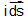on element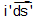), in modern symbols: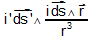Intensity of the force:     i i' ds ds' sinα cosβ      (β being the angle between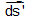and plane P).

This force, whose expression does not assign a symmetric role to the two elements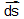and, is always perpendicular to the element.

### The 1980s: Ampère's formula, a new scientific challenge?

Since the 1980s, a certain number of physicists - supported by theoretical work as well as by experimental results - have believed that one must bring back from oblivion Ampère's original formula, which now finds itself nearly two centuries after its “invention” the subject of scientific controversy.

Some even claim that several experiments can be explained only by Ampère's formula.

Are we returning to an archaic past, rendered outdated by the electromagnetism of Maxwell-Lorentz? Did Ampère's formula simply fall out of use, lapsed without being challenged, dethroned by a theory that was more fruitful, more aesthetic, and easier to apply, or is it incompatible with modern electromagnetism, which explains a great number of phenomena?

### The ambiguity of Maxwell's judgment

While Maxwell considered Ampère's formula to be “fundamental”, he nonetheless added:

“Hence no statement about the mutual action of two elements of circuits can be said to rest on purely experimental grounds.” (A Treatise..., vol. 2, p. 163)

Indeed, as electric currents form closed circuits, it is impossible to isolate physically the action of a current element (we are not considering here open circuits as a radio antenna, seat of high-frequency electrical oscillations). While many experimental tricks allow one to make a small portion of a circuit (C') mobile, this portion is subject to the action of the entire circuit (C), but one cannot isolate the action of a single one of circuit (C) elements.

An infinity of elementary formulas, including those of Ampère and Grassmann, can lead to the same force exerted on element i'ds' by integration over the totality of the circuit (C).

Why, then, does the same Maxwell describe Ampère's formula as a “fundamental formula of electrodynamics”? Indeed it is the only one that respects the Newtonian principle of action and reaction. In general Grassmann's force is not carried along the straight line that joins two elements. In addition, Grassmann's forceexerted by the elementon elementdoes not have the same intensity as that exerted byon, obtained by reversing the roles of the two elements.

As the physicist R.A.R. Tricker has emphasized, today we consider Newton's principle as applying only to finite forces, having a physical reality, therefore to the forces between circuits:

“The reader may well take the view that questions which, even in principle, cannot be put to the test of experience [such as the correct elemental formula], do not belong to science, but in spite of this Ampère has been subjected to considerable criticism. Curiously enough, the one criticism to which he would be vulnerable, [was] namely that he, too, along with most of his critics, thought that he was arriving at the law of force which current elements actually exerted upon each other...” (R.A.R. Tricker, Early Electrodynamics, 1965, p. 99)

### Ampère's formula dethroned by Maxwell's equations and “Laplace's force”

By integrating Grassmann's forceover the circuit (C), one obtains the force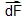exerted by this circuit (C) on the elementof circuit (C'):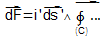The result of the summation over (C) subsequently was identified as being the magnetic field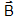created by circuit (C). Grassman's force thus takes the simple vector form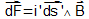. This force is perpendicular toand to, and its intensity is i'ds'B sinε, where ε is the angle betweenand. In France, at least, this law is known as "Laplace force".

 Laplace force:Ampère had established an equivalent law [See the page In Search of...]. Indeed, he showed that the force exerted by a closed circuit on element ds' was perpendicular to this element and to a straight line that he called the directrice. He expressed the intensity of this force as:
½ D i i' ds' sinε.
One can recognize in the factor ½ Di, associated with the directrice, the characteristics of the magnetic field B. But, Ampère saw in this law only a mathematical consequence of an action at a distance between current elements.

The triumph of Maxwell's theory, based on the continuous propagation of electromagnetic actions, explains the abandonment of Ampère's formula in favor of Grassmann's which fits perfectly into the Maxwellian framework, because it is easy, as we have seen, to link it to the field. Maxwell's homage to the historical Ampère's formula is thus somewhat paradoxical.

### Repulsive forces between collinear elements?

A question remains open. Could one, contrary to what Maxwell asserts, decide between the elementary formulas of Ampère and Grassmann by experiment? And if experiment decided in favor of Ampère's force, would that challenge the edifice erected by Maxwell, itself inextricably linked to the theory of relativity?

Ampère's formula implies, in contrast to that of Grassmann, the existence of a repulsive force between two collinear current elements. Indeed, if the two elements are collinear, the angles α and β (Fig. 1) are zero and the force between ds and ds' becomes:
- ½ i i' ds ds'/ r2.
The force being negative, it is a repulsion. In contrast, Grassmann's formula, dF = i i' ds ds' sinα cosθ, implies that the force between collinear ds and ds' is zero.
According to Ampère, in a metal wire carrying a current, there should be a repulsive force between two consecutive elements. So, could we decide between the two formulas by studying the action exerted between two parts of the same circuit?

### Ampère's "hairpin experiment" revisited in the 1980s

In 1822, Ampere thought he held a proof in favor of his formula with his experiment of the floating conductor, sometimes called the "hairpin experiment". [See the video L'expérience du conducteur flottanton the page In Search of...].Fig. 2. A copper wire nqr, in the shape of a hairpin, insulated except for the bare ends n and r, floats on the surface of the mercury contained in two sections of a circular container. When a strong current flows through the circuit, the hairpin wire moves away from the fixed contacts s and m regardless of the direction of the current.

During the 1980s, several researchers revisited this experiment.

- Peter Graneau at MIT observed in 1981, with currents of several hundred amperes, strong turbulence in the mercury when the movement of the hairpin was blocked. This turbulence seemed to manifest the repulsion undergone by the portion of mercury carrying the current

 - Panos T. Pappas in 1983 replaced Ampère's hairpin with a wire formed into a rectangle lacking one of its shorter sides and suspended in a way to constitute a pendulum (Fig. 3). The author determined the amount of motion communicated to the pendulum, and he attributed this motion to Ampère's repulsive force. This experiment was executed with greater precision by Peter and Neal Graneau (1986) using the discharge of a series of capacitors. Fig. 3. The principle of Pappas' impulse pendulum.The ends of the movable conductor are in electrical contact with the fixed terminals of a battery via the small cups of mercury B and E. When the current is turned on, the pendulum undergoes a repulsion and electrical contact is broken.- Still in line with Ampère's experiment, other physicists have sought to “weigh” the possible repulsive force. Thus Peter Graneau in 1986, then Rémi Saumont in 1992, suspended the same type of mobile conductor in the form of an incomplete rectangle vertically under the pan of a precision balance. The vertical repulsive force that it underwent from the fixed part of the circuit then could be measured.

In fact the different variations of Ampère's experiment are interpreted in the context of modern physics by using the Laplace force, and therefore the elemental force of Grassmann. Indeed the transverse part of incomplete rectangles is subject to a Laplacian force under the action of the magnetic field created by the circuit. This force is perpendicular to the transverse portion, and therefore tends to separate the movable part from the rest of the circuit.

Only the calculation of the intensity of the force experienced by the movable part would be likely to decide between the two basic laws. But, can one calculate, by integrating Ampère's formula, the repulsive force between two parts of the same circuit? If one likens the conductor to a line without thickness, divisible into “infinitely short” current elements, integration leads to an infinite force between two adjacent collinear elements.

In contrast, several authors have proposed calculating methods that better take into account the actual structure of conductors: a current element is not infinitely short and the wire diameter is not zero.

 - For Graneau, the size of the current element cannot be smaller than that of the unit cell of the metallic lattice, of the order of a nanometer. Computers performed numerical integrations by summation over a large number of small elements but the size of these elements remains much larger than a nanometer. By extrapolating from the results obtained, it is hoped to obtain an order of magnitude of the force [Graneau, 1986]. Fig. 4. The principle of calculation by summation over finite elements [Graneau, 1986]. When one divides a conductor into smaller and smaller elements, the force calculated by this method of approximation increases, but less and less rapidly as one approaches atomic magnitudes.- Other writers, taking into account a volumetric distribution of the current in a conductor with a non-zero diameter, avoid choosing numerical methods and perform integrations. They therefore must deal with sextuple integrals [Marcelo Bueno and A. K. T. Assis, 1996].
- Finally, calculations can be performed more easily within the framework of classical electromagnetism using the expression for the inductance of the circuit, a notion that came along well after Ampère [R.A.R. Tricker, 1965, or Bueno and Assis, 1998].

Regardless of the calculating method chosen, the force exerted by a circuit on one of its elements has a value low enough so that the mechanical stresses remain imperceptible in solid conductors, at least as long as the current has not an unusually high intensity. But it can explain the observed effects in circuits with liquid or movable part, as in the previous experiments.

Some authors conclude with the triumph of Ampère's formula. According to them, integrations based on Grassmann's formula lead to clearly weaker forces. However, in 1996, Assis and Bueno corrected an error in the reasoning underlying these calculations and achieved, using Grassmann's formula, the same results as with Ampère's formula. In 1998, they also showed that the inductance method constituted an indirect proof of the equivalence between the formulas of Ampère and Grassmann.

Consequently it does not seem possible to decide between the formulas of Ampère and Grassmann by experiments of Ampère's type.

### Other experiments enter the controversy

The previous experiments considered the overall force exerted by a circuit on one of its parts. While the formulas of Grassmann and Ampère give the same result for this overall force, they may not be equivalent for the distribution of force along the circuit. In order to test this hypotheses, some tried to highlight local effects of longitudinal forces, effects for which only Ampère's force could account.

- Inspired by an experiment performed by Neumann for his students during the 1880s, Graneau placed two short copper rods end to end in a rectilinear narrow channel filled with mercury. When this channel carried a current of 450 amps, the two rods repelled each other.

 Fig. 5. In the middle of a long channel of mercury, two copper rods AB and CD are placed end to end. When current flows, the rods repel each other. (The rods are covered with insulation, and the conductivity of copper is much greater than that of mercury, which explains the patternin blackof the lines of current). Graneau, 1986.- In the early 1960s, Jan Nasilovski already had noticed that a very strong flow of current caused the explosion of conducting wires into a series of fragments (Fig. 6). Graneau repeated the experiment in the 1980s with currents of more than 5,000 amps but of short enough duration to keep the temperature well below the metal's melting point. Examination of the microscopic structure of the fracture regions seemed to confirm a mechanical cause. The fractures, therefore, could be due to Ampère's force.Fig. 6. Fragments resulting from the explosion of conductors [Jan Nasilowski].
 - In another experiment [Linda J. Ruscak and R. N. Bruce, 1987] a copper rod one meter long was cut into segments of one centimeter and stacked in a glass tube. Springs held them firmly in contact with each other. Current pulses ranging from 3 to 30 kiloamps caused them to separate, while electric arcs leaped in the gaps. Fig. 7. Separation and formation of arcs between copper segments in the experiment of Ruscak and Bruce.- One will add an experiment carried out after the observation of a paradoxical effect during experiments on the “electromagnetic railgun” for the U.S. Strategic Defense Initiative. The left part of Figure 8 shows the very simple principle of the railgun or "cannon". When a very intense current flows in the circuit consisting of two long horizontal rails and a movable bar placed on these rails, the bar starts to move. The imparting of motion to the projectile is explained very classically by the Laplace force experienced by the movable bar in the circuit's own magnetic field. In 1984, with a peak intensity of more than 2 million amps, it was possible to drive an object weighing 300g at a speed of 15,000 km/h. During this experiment, it was observed that the rails had undergone unexpected mechanical deformations. Graneau then modified the experiment (Fig. 8, on the right) by blocking the crossbar. Pulses of 100 kiloamps were enough to cause deformations in the thinnest parts of the rails which he attributed to longitudinal Ampère forces.Fig. 8 : The “electromagnetic railgun”. In Graneau's version (at right) the crossbar is blocked.

All these experiments were presented as proofs for the existence of longitudinal forces, but alternative interpretations could be put forward as well.

Other experiments aimed at showing longitudinal forces in liquid metals and in plasmas. Although some were very dramatic, we will not mention them here because they are more easily subjected to alternative interpretations.

### Longitudinal forces and modern electromagnetism

Longitudinal forces in conductors are absent from most books on electromagnetism. Additionally, their potential effects occur only with unusually high current intensities. Nonetheless, according to some authors, Maxwell's electromagnetism does not exclude the existence of longitudinal stresses that may play a role in the experiments presented above. These stresses arise from the application to conductors of Maxwell's theory of “stress tensors”, entirely unconnected to Ampère's force.

### The Lorentz force and Ampère force: A contradiction?

Maxwell's equations deal only with electromagnetic field and do not include a force law. For Maxwell, the formulas of Grassmann and Ampère were compatible with his theory, even though he expressed a preference for that of Ampère.

Later, Lorentz expressed the force acting on charged particle q in a vacuum in the presence of magnetic field:

 Lorentz force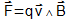It is henceforth the Lorentz force, remarkably verified by experiment, that classic accounts of electromagnetism adopt as an elementary law of force. At any time this force is perpendicular to the direction of the motion of the particle. Thus the current element constituted by a charged particle in motion undergoes a force that, like that of Grassmann, has no longitudinal component.

The expression of the Laplace force,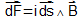, is usually presented as a consequence of that of Lorentz. In the models proposed to account for conduction in metals, everything happens as if the forces experienced by the conduction electrons were transmitted to the metallic lattice. The force being perpendicular to the direction of the electrons movement, a metallic conductor element can undergo only transversal forces. The remarkable accuracy of the verifications of the Lorentz law thus would justify abandoning Ampère's formula.

Nonetheless, the justification of Laplace's law by using the Lorentz force has been challenged. A metal conductor is not comparable to an electron beam in a vacuum. We must take into account the physical nature of the current element consisting of positive ions and electrons. According to M. Rambaut and J. P. Vigier, the application of the Maxwell-Lorentz-Einstein laws of electromagnetism to the collective behavior of this ensemble of charged particles leads, in nonrelativistic approximation, to Ampère's formula (1989). Currents in metals would obey Ampère's law, while currents in a vacuum would obey Grassmann's law.

### Action at a distance versus field theory and Einsteinian relativity

Still, while modern electromagnetism predicts - and in a simpler way - the same effects as Ampère's formula, why argue - as some continue to do - for the “rehabilitation” of this formula? Is there still room for controversy?

The challenge is actually theoretical. The Ampère force is a force of instantaneous action at a distance. In this respect it is fundamentally inconsistent with Maxwell's theory based on the continuous propagation of electromagnetic action.

The triumph of Maxwell's theory is based on its extraordinary fertility. His equations account for, among other things, the propagation of electromagnetic waves at the speed c = 300,000 km/s. The electromagnetic field, which allows the transport of energy, exists in a vacuum devoid of charges. Furthermore, it is Maxwell's theory that “laid down the conditions” in some way for Einstein theory of relativity. The edifice of Maxwell-Lorentz electromagnetism and Einsteinian relativity is thus an apparently incontestable pillar of all twentieth-century physics.

Before Maxwell, physicists such as Weber and then Neumann developed an electrodynamics derived from Ampère's formula. They sought a law of force or of potential, between current elements or between moving electric charges, that would account for all phenomena including the phenomenon of induction - which Ampère's formula did not do. Thus, Weber proposed an elementary force between electric charges consisting of three terms dependant on the distance, the velocity, and the acceleration of one charge relative to the other. The first term is nothing more than Coulomb's law, the second can be deduced from Ampère's formula, while the third accounts for the phenomenon of induction.

Several of the contemporary defenders of Ampère's force pursue the “weberian” electrodynamics project as an alternative to that of Maxwell (A. K. T. Assis, Weber's Electrodynamics, 1994 or P. and N. Graneau, Newtonian electrodynamics, 1996). The reference to Newton, like references to Ampère or to his successors, is indicative of a project that goes beyond the field of electricity. It is a matter of building a physics based on interactions at a distance between material elements. One could object that Newton or Ampère themselves were far from considering instantaneous action at a distance as the last word in physics. Behind their mathematical laws of gravitation or electrodynamics, which involve only interacting elements and their distance, both were convinced of the existence of a deeper reality based on a continuous transmission in the intermediate space [Seethe page Des théories mathématiquement équivalentes, physiquement différentes]. However, that does not prevent attempts to account for phenomena customarily described by means of Maxwell's theory using Weber's electrodynamics: radiation effects, the propagation of light... “A lot of theoretical and experimental work will be necessary in this direction,” wrote Assis in 1989.

The idea of applying a law analogous to that of Weber for electromagnetism (involving several terms) to gravitation dates back to the 1870s and was considered by Weber himself. One adds to Newton's force of gravitation some terms dependent on the relative speed of masses, on the model of terms added by Weber to the electric force of Coulomb. According to Assis [Relational Mechanics, 1999], this weberian mechanics allows the deduction of the first two Newton's laws of dynamics,- the principle of inertia and the fundamental relationship of dynamics,- and to establish the proportionality between inert and gravitational mass. The precession of the perihelion of the planets can be calculated without recourse to the equations of general relativity...

### A widespread dissidence that may inspire suspicion?

When one examines the publications of certain supporters of the rehabilitation of Ampère's force, one sometimes finds an opposition to the mainstream establishment - “official science” - that is far from being limited to Maxwell's electromagnetism. Someone denounces “the scam of special relativity” or proposes to take advantage of “the infinite energy of the vacuum”. Another counters the standard Big Bang theory with that of a stationary universe. The red shift of light from distant galaxies, usually interpreted by the expansion of the universe, would be explained by a theory of “light fatigue”. One also finds articles on cold fusion, etc..

These connections can lead to a certain degree of suspicion. However, one will recall that certain beliefs of Ampère, without mentioning his interest in animal magnetism, reeked of heresy to numerous members of the Academy. Certainly, there is hardly any experimental reason for doubting Maxwell's electromagnetism, and it can seem that working to build an alternative electrodynamics is wasting one's energy for nothing. Yet, can one not approve of the Scientific American review of Graneau's book, Newtonian Electrodynamics: "Highly recommended to the open-minded, and to any who agree with Maxwell about the prudential' role of pluralism in ensuring the health of science and, indeed, in ensuring its life." ?”